# Introduction to Types of TrianglesHi, and welcome to this review of different types of triangles! Before we begin, here’s a review of the basics.

A triangle has three straight sides that connect. The length of the sides can vary but the length of the largest side can’t be equal or greater to the sum of the other two sides. In addition, a triangle has three interior angles, and the sum of those three angles is always 180 degrees. This is true for all triangles, including the six types we’re looking at today.

## Different Types of Triangles

We’re going to break our six types of triangles into two groups of three.

Let’s start with the three types of triangles that are categorized by the measure of their largest angle. These are the acute, right, and obtuse triangles.But how do you know which is which? Take a look at the largest angle of each triangle and note whether or not the angle is more than, less than, or equal to 90 degrees.

### Acute Triangle

We can see that the largest angle in the triangle on the left is 70 degrees. 70 is less than 90, so this is an acute triangle. Just remember that acute angles are less than 90 degrees. This one is easy to remember, since “cute” things are often small, like puppies and kittens.

### Right Triangle

We can see that in the middle triangle the largest angle is exactly 90 degrees. You might remember that a 90-degree angle is a right angle, so this triangle is a right triangle.

### Obtuse Triangle

Finally, in the triangle on the right, the largest angle is 117 degrees. Because this is more than 90 degrees, this is an obtuse angle, so we call this triangle an obtuse triangle.That’s all there is to it for these three types! We just find the largest angle and the name of the triangle will correspond to the name of that angle.

Our second set of triangles is categorized by how many of the sides have the same length. Here are three triangles with the lengths of the sides included:### Equilateral Triangle

In the triangle on the left, we can see that all three sides are the same length and measure 9 centimeters. A triangle like this one where all the sides are the same is called an equilateral triangle. It’s not too tough of a name to remember since the beginning of equilateral sounds like the word equal, and the word lateral means “side.”

### Isosceles Triangle

In the middle triangle, we can see that two of the sides are the same length and measure 8 cm while the third is 9 cm. When two of the sides of a triangle are the same it’s called an isosceles triangle. It’s a hard one to spell, but an easy one to recognize!

### Scalene Triangle

In our last triangle, none of the sides have the same length, so this is called a scalene triangle.While you often see these three types of triangles identified by the lengths of their sides, they can also be categorized by their angles. It works exactly the same way:When all the angles are the same 60 degrees, it’s an equilateral triangle. Technically, it’s called an equiangular triangle but they’re exactly the same thing because all equilateral triangles are also equiangular triangles. When two of the angles are the same it’s an isosceles triangle. And when none of the angles are the same it’s a scalene triangle.

So far, we’ve covered six types of triangles, which is technically all of the types, but we’re not done yet. If we wanted to be really specific when naming our triangles, we can combine names from each group; one from the first group and one from the second group.For instance, the triangle above is an acute scalene triangle. Its largest angle is less than 90 degrees, so it’s acute, and none of its angles are the same, so it is scalene.

Before we go, how about you give it a try. Identify these two triangles by choosing either acute, right, or obtuse and then choosing equilateral, isosceles, or scalene.Think you got it? The triangle on the left is an obtuse scalene triangle, while the one on the right is a right isosceles triangle.I hope this review was helpful! Thanks for watching, and happy studying!

### What are the six types of triangles?

#### A

The six types of triangles are: isosceles, equilateral, scalene, obtuse, acute, and right.

An isosceles triangle is a triangle with two congruent sides and one unique side and angle.
Ex.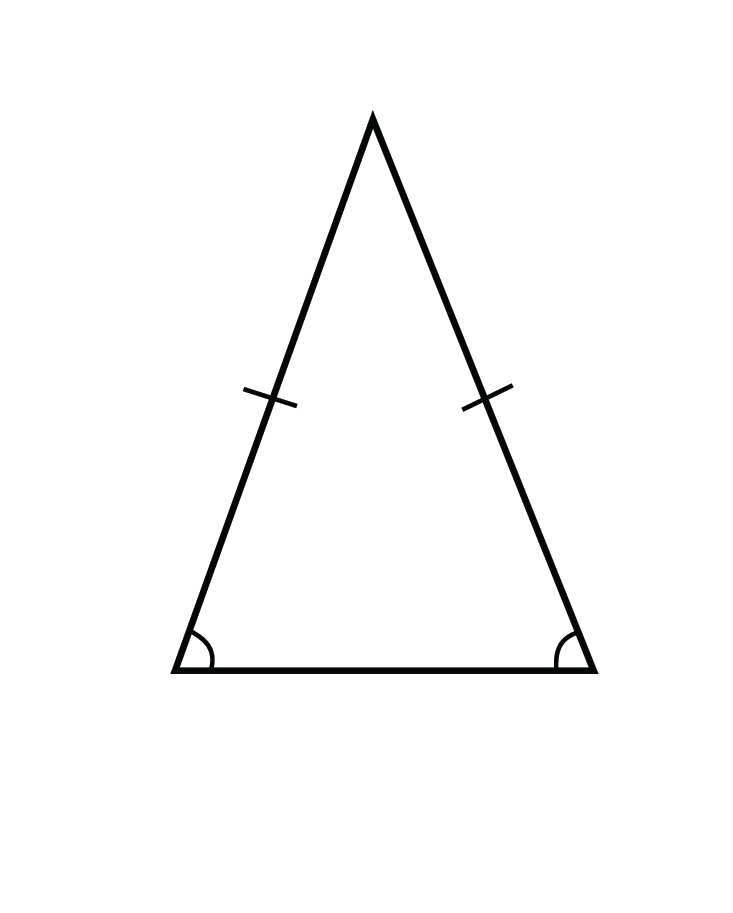An equilateral triangle is a triangle with three congruent sides and three congruent angles.
Ex.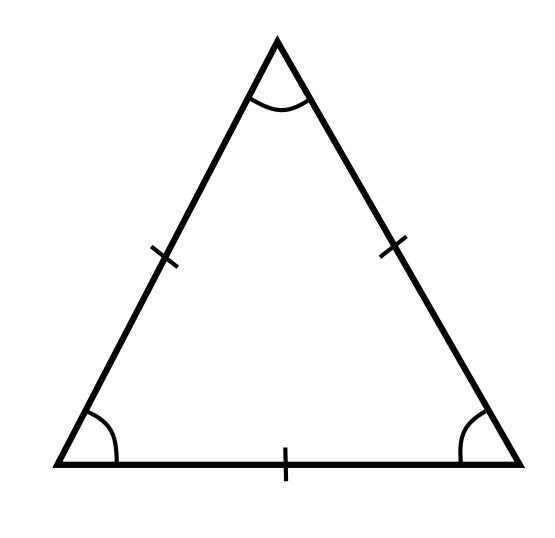A scalene triangle is a triangle with no congruent sides and no congruent angles.
Ex.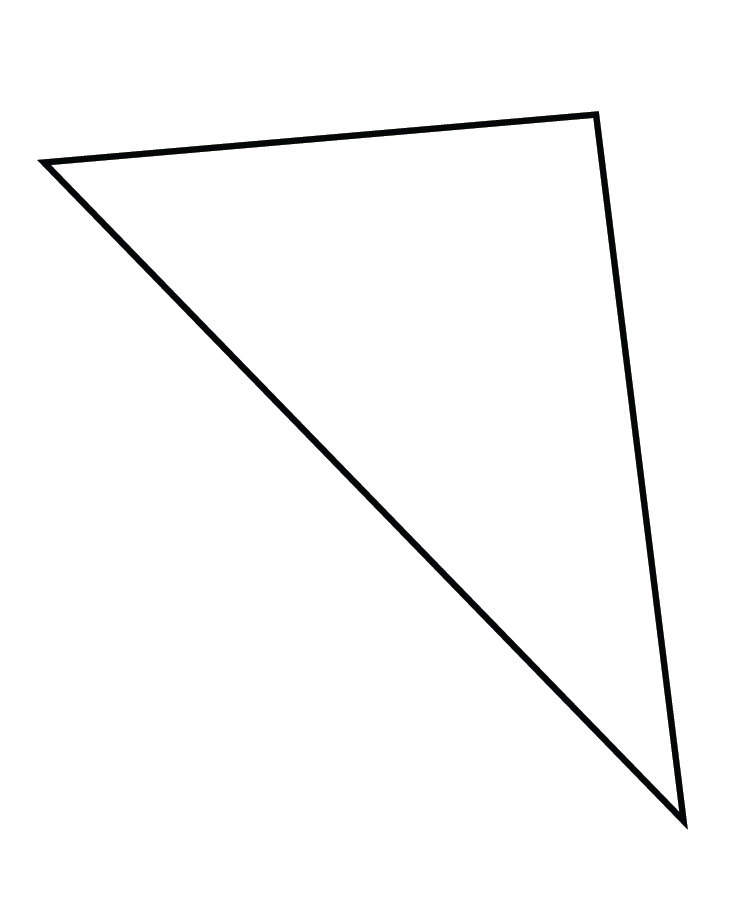An obtuse triangle is a triangle that has an obtuse angle.
Ex.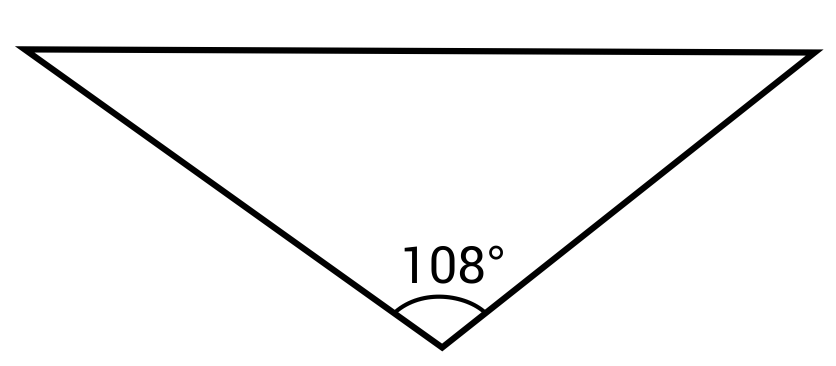An acute triangle is a triangle that has three acute angles.
Ex.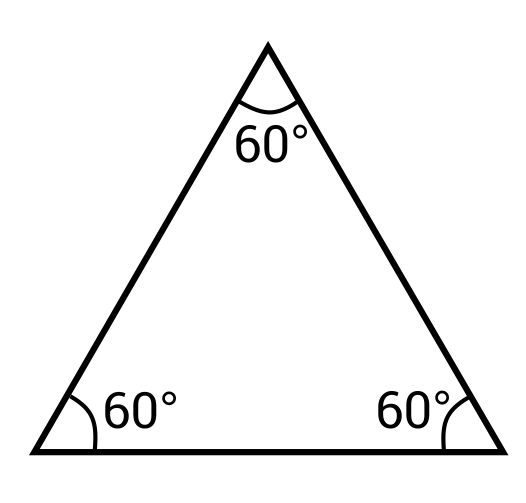A right triangle is a triangle that has one right angle.
Ex.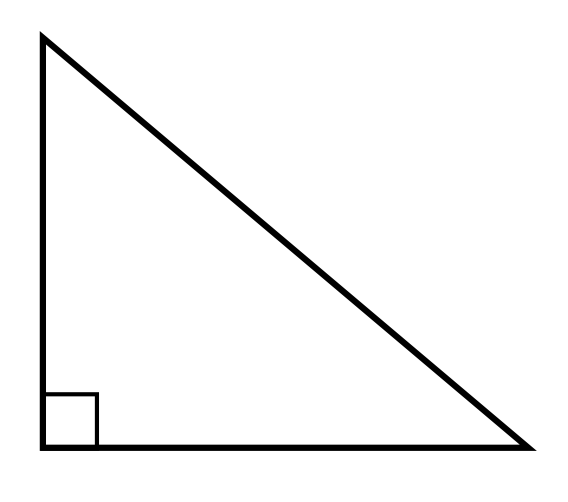### What are properties of a scalene triangle?

#### A

Scalene triangles are triangles that have three unique side lengths and three unique angle measures.

### What is scalene and right?

#### A

A scalene triangle is a triangle that has no equal sides or angles. A right triangle is a triangle that has one right (90°) angle. A triangle can be scalene and right if one of its unique angle measures is 90°.
Ex.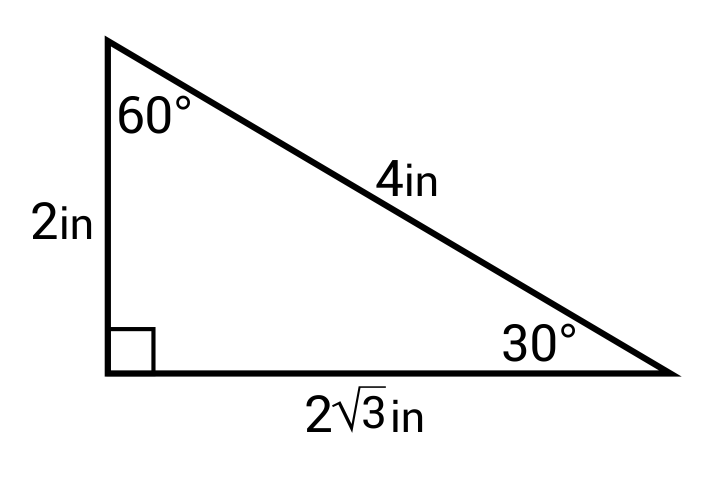### How many sides are equal in an isosceles triangle?

#### A

An isosceles triangle has two equal sides and two equal angles.

### What is a true triangle?

#### A

A true triangle is a shape that has three sides and three angles. The lengths of two of the sides must add up to a number greater than the third side, and the three angles must add up to 180°.

### Which angles are equal in an isosceles triangle?

#### A

The two angles that are equal in an isosceles triangle are the two base angles. An isosceles triangle has two equal sides that meet at a point. The angle made by these two lines meeting is the unique angle. The angles formed by the bases of these lines and a third, unique side, are the two equal angles, called base angles.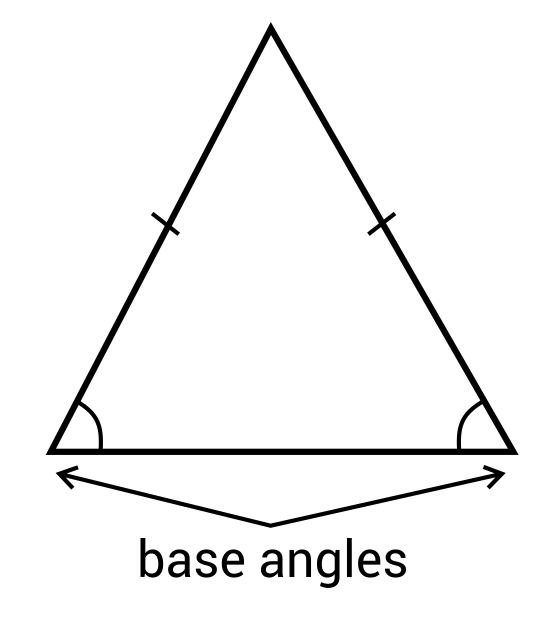### What are the characteristics of an equilateral triangle?

#### A

An equilateral triangle is a triangle that has three equal sides and three equal angles.

## Types of Triangles by Shapes and Names PDF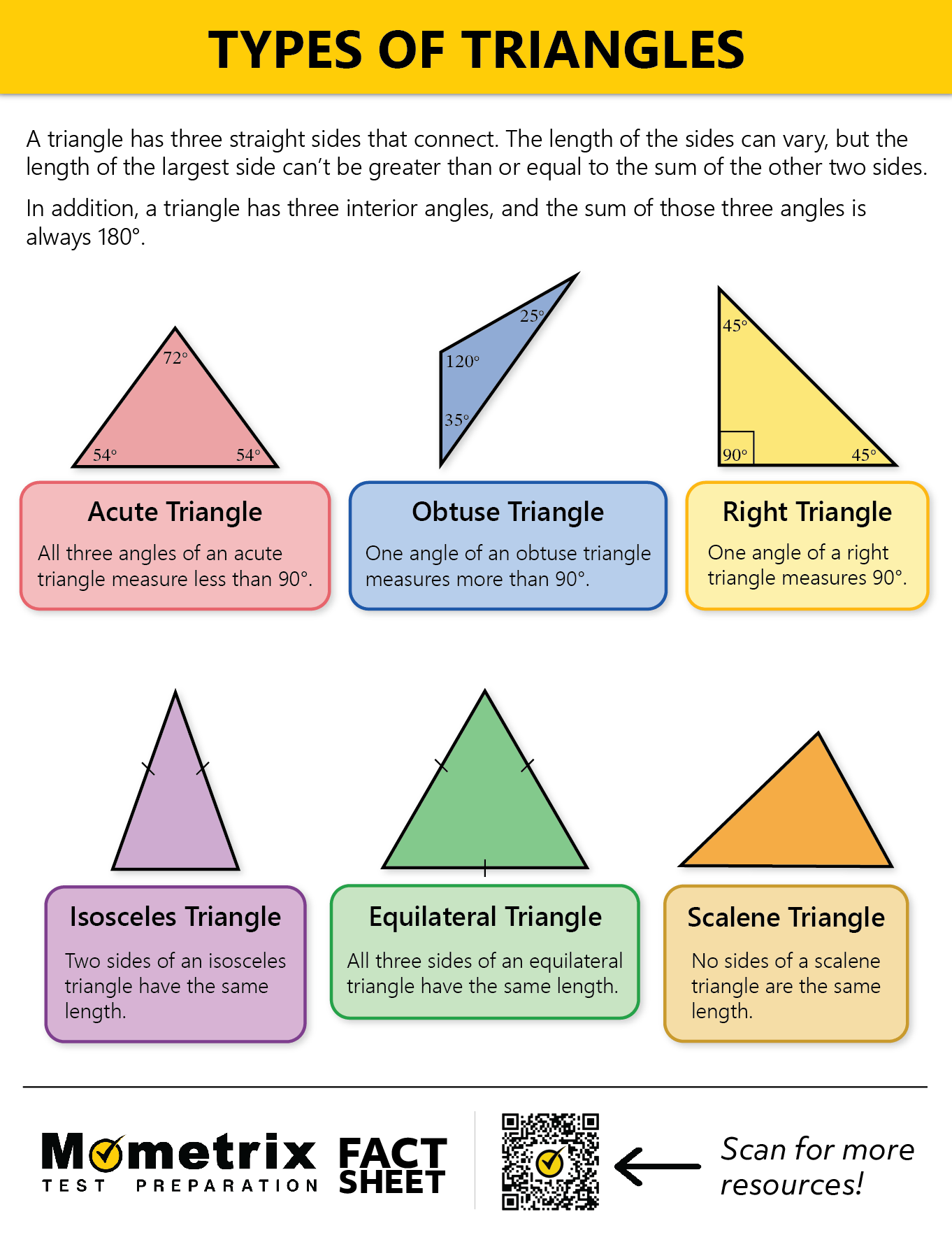## Practice Questions

Question #1:

What kind of triangle is this?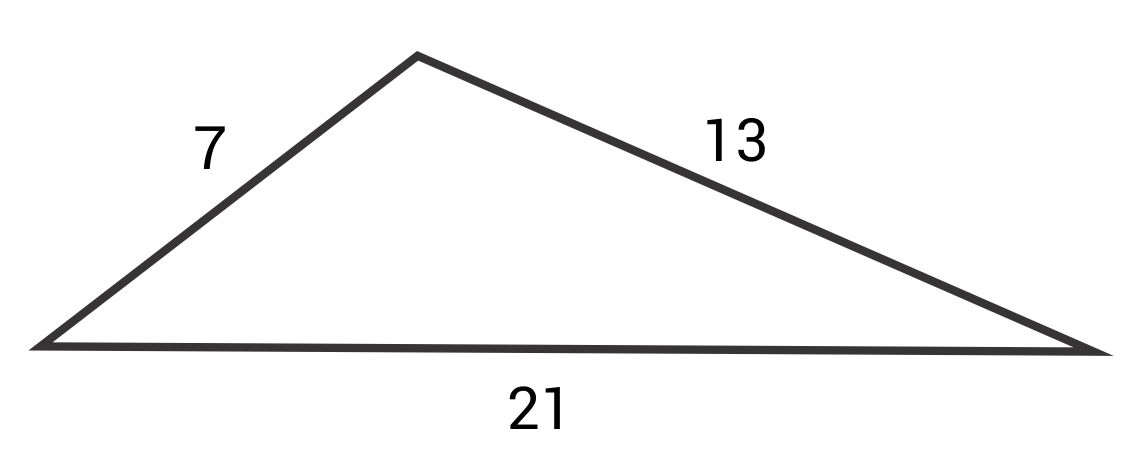Acute

Equilateral

Isosceles

Scalene

The correct answer is scalene. A scalene triangle is a triangle with no equivalent sides.

Question #2:

What kind of triangle is this?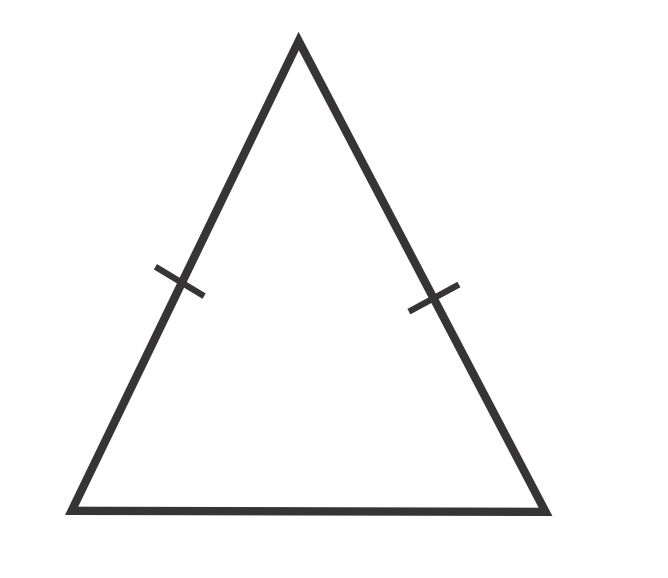Obtuse isosceles

Acute isosceles

Acute scalene

Obtuse scalene

The correct answer is acute isosceles. An isosceles triangle has two congruent sides (as shown by the two tick marks) and one unique side. It is acute because the unique angle (the one on top) is less than 90°.

Question #3:

What kind of triangle is this?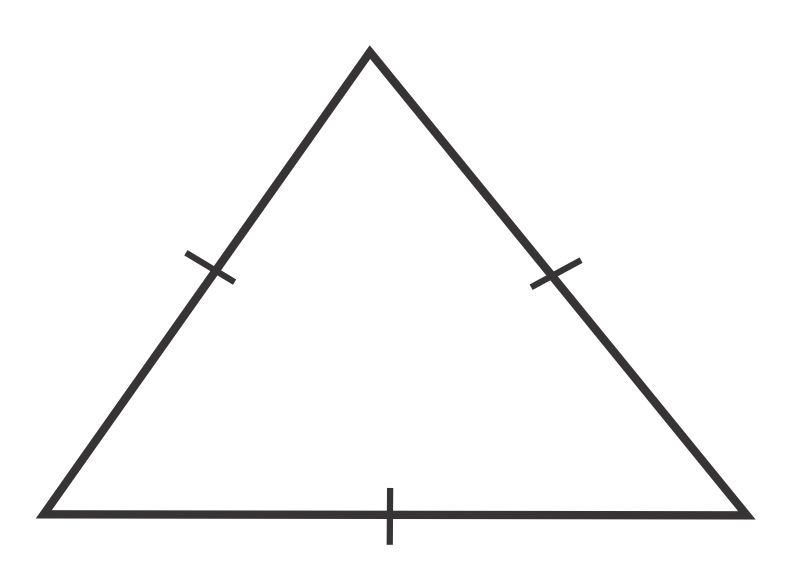Acute

Obtuse

Equilateral

Scalene

The correct answer is equilateral. An equilateral triangle is a triangle with three congruent sides and three congruent angles.

Question #4:

What kind of triangle is this?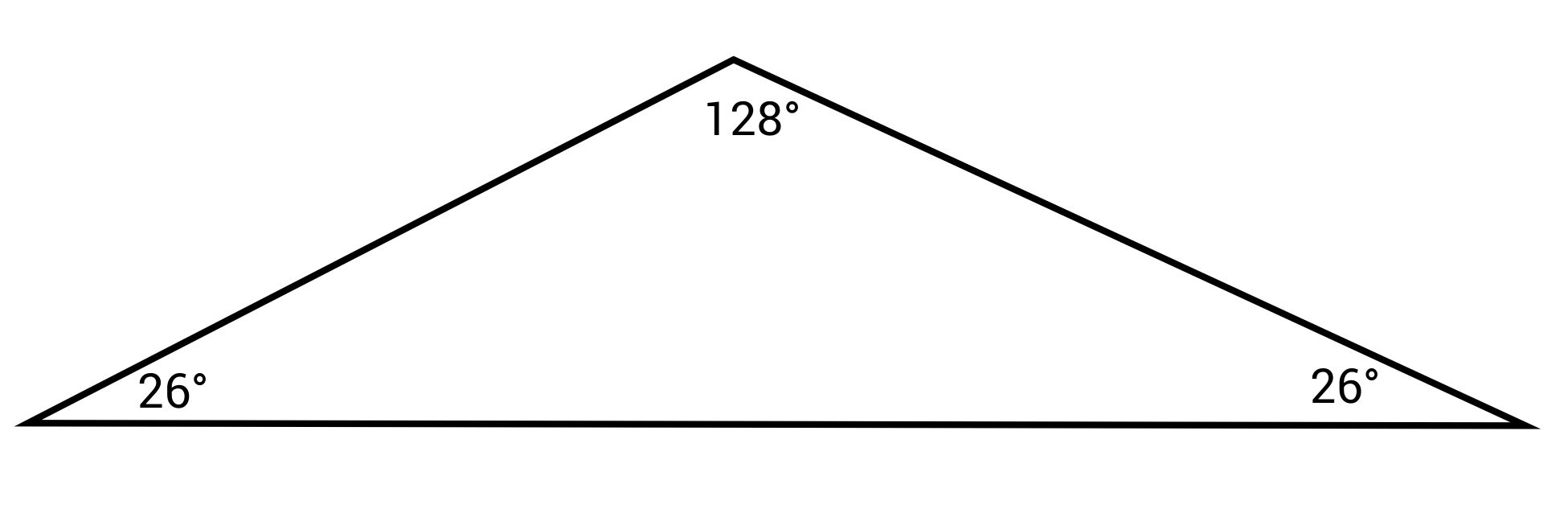Acute isosceles

Acute scalene

Obtuse isosceles

Obtuse scalene

The correct answer is obtuse isosceles. An isosceles triangle is one with two congruent sides and two congruent angles and one unique side and angle. This triangle has two congruent angles on the bottom. It is obtuse because the unique angle is greater than 90°.

Question #5:

What kind of triangle is this?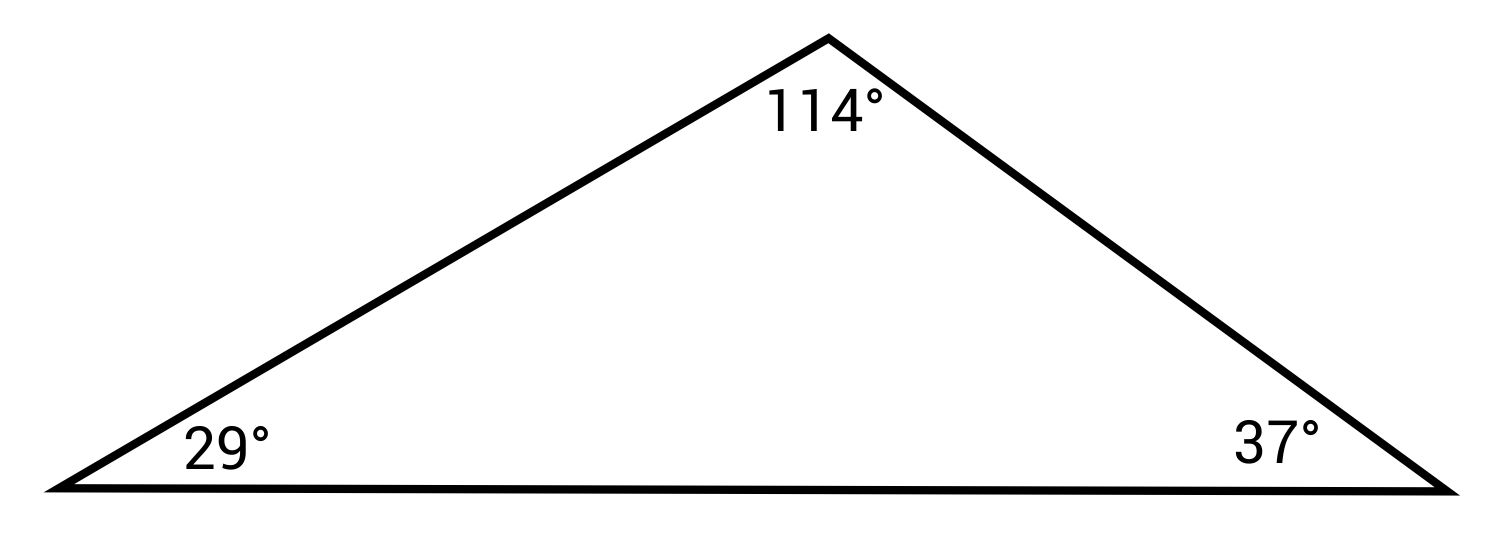Acute scalene

Obtuse scalene

Acute isosceles

Obtuse isosceles

The correct answer is obtuse scalene. A scalene triangle is one with three unique side lengths and angle measures. It is obtuse because the largest angle is greater than 90°.

511711

by Mometrix Test Preparation | This Page Last Updated: November 21, 2023

Get Actionable Study Tips
Join our newsletter to get the study tips, test-taking strategies, and key insights that high-performing students use.
Get Actionable Study Tips
Join our newsletter to get the study tips, test-taking strategies, and key insights that high-performing students use!